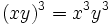# Cube map is endomorphism iff abelian (if order is not a multiple of 3)

This article describes an easy-to-prove fact about basic notions in group theory, that is not very well-known or important in itself
View other elementary non-basic facts
VIEW FACTS USING THIS: directly | directly or indirectly, upto two steps | directly or indirectly, upto three steps|

## Statement

### Verbal statement

Consider a finite group whose order is not a multiple of 3. Then, the Cube map (?) (viz the map sending each element of the group to its cube) is an endomorphism if and only if the group is abelian.

### Statement with symbols

Let$G$ be a finite group whose order is not a multiple of 3. Then, the map$\sigma$ defined as$\sigma(x) = x^3$ is an endomorphism if and only if$G$ is abelian.

## Related facts

We say that a group is a n-abelian group if the$n^{th}$ power map is an endomorphism. Here are some related facts about$n$-abelian groups.

Value of$n$ (note that the condition for$n$ is the same as the condition for$1-n$) Characterization of$n$-abelian groups Proof Other related facts
0 all groups obvious
1 all groups obvious
2 abelian groups only 2-abelian iff abelian endomorphism sends more than three-fourths of elements to squares implies abelian
-1 abelian groups only -1-abelian iff abelian
3 3-abelian group means: 2-Engel group and derived subgroup has exponent dividing three Levi's characterization of 3-abelian groups cube map is surjective endomorphism implies abelian, cube map is endomorphism iff abelian (if order is not a multiple of 3), cube map is endomorphism implies class three
-2 same as for 3-abelian (based on n-abelian iff (1-n)-abelian)

## Facts used

1. Abelian implies universal power map is endomorphism: In an abelian group, the$n^{th}$ power map is an endomorphism for all$n$.
2. Cube map is surjective endomorphism implies abelian
3. kth power map is bijective iff k is relatively prime to the order

## Proof

### Abelian implies cube map is endomorphism

This is a direct consequence of fact (1).

### Cube map is endomorphism implies abelian

Given: A finite group$G$ whose order is relatively prime to$3$, and such that$x \mapsto x^3$ is an endomorphism of$G$.

To prove:$G$ is abelian.

Proof:

Step no. Assertion Given data used Facts used Previous steps used Explanation
1 The cube map is an automorphism, and in particular, a surjective endomorphism, of$G$. The order is not a multiple of 3, and the cube map is an endomorphism. Fact (3) -- Since the order is not a multiple of$3$, the order is relatively prime to$3$, so fact (3) yields that the cube map is bijective. Since we already know that the cube map is an endomorphism, this yields that the cube map is an automorphism of$G$.
2$G$ is abelian. -- Fact (2) Step (1) Since the cube map is an automorphism,$G$ must be abelian.

### Difference from the corresponding statement for the square map

In the case of the square map, we prove something much stronger:$(xy)^2 = x^2y^2 \iff xy = yx$

In the case of the cube map, this is no longer true. That is, it may so happen that$(xy)^3 = x^3y^3$ although$xy \ne yx$. Thus, to show that$xy = yx$ we need to not only use that$(xy)^3 = x^3y^3$ but also use that this identity is valid for other elements picked from$G$ (specifically, that it is valid for their cuberoots).